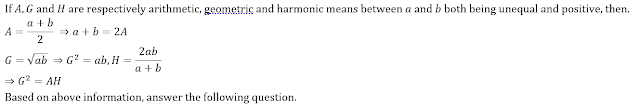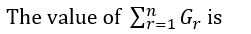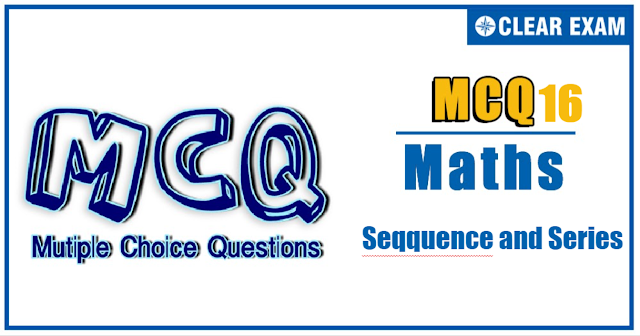## [LATEST]\$type=sticky\$show=home\$rm=0\$va=0\$count=4\$va=0

The unit Sequence and Series holds sheer significance in the JEE Advanced and other engineering exams. It has a weightage of 8%. With focused practice good marks can be fetched from this section. These questions are important in achieving your success in JEE and Other Engineering Exams..

Q1.If the geometric and harmonic means of two numbers are 16 12 4/5, then the ratio of one number to the other is
•  1:4
•  2:3
•  1:2
•  2:1
Q2.  Sum of certain consecutive odd positive integers is 572-132
Number of integers are
•  40
•  37
•  44
•  51
Q3.  Consider three distinct real numbers a,b,c in a G.P. with a2+b2+c2=t2 and a+b+c=αt. Sum of the common ratio and its reciprocal is denoted by S
Complete set of a2 is
•   (1/3,3)
•   [1/3,3]
•   (1/3,3)-{1}
•   (-∞,1/3)∪(3,∞)

Q4.  In a G.P., the sum of the first and last term is 66, the product of the second and the last but one is 128 and the sum of the terms is 126
If an increasing G.P. is considered, then the number of terms in G.P. is
•  9
•  8
•  12
•  6
Q5.  Four different integers form an increasing A.P. One of these numbers is equal to the sum of the squares of the other three numbers. Then
The product of all numbers is
•  -2
•  1
•  0
•  2
Q6.  Consider the sequence in the form of groups (1), (2, 2), (3, 3, 3), (4, 4, 4, 4), (5, 5, 5, 5), …
The 2000th term of the sequence is not divisible by
•  3
•  9
•  7
•  None of These
Q7.  There are two sets A and B each of which consists of three numbers in A.P. whose sum is 15 and where D and d are the common differences such that D-d=1. If p/q=7/8, where p and q are the product of the numbers, respectively, and d>0 in the two sets
Sum of the product of the numbers in set A taken two at a time is
•  51
•  71
•  74
•  86
Q8.  Let A_1,A_2,A_3,…,A_m be the arithmetic means between -2 and 1027 and G_1,G_2,G_3,…,G_n be the geometric means between 1 and 1024. The product of geometric means is 245and sum of arithmetic means is 1025×171•  512
•  2046
•  1022
•  None of these
Q9.  Two consecutive numbers from 1,2,3,…,n are removed. The arithmetic mean of the remaining numbers is 105/4
The value of n lies in
•   [45, 55]
•   [52, 60]
•   [41, 49]
•  None of These
Q10.  Two arithmetic progressions have the same numbers. The ratio of the last term of the first progression to first term of the second progression is equal to the ratio of the last term of the second progression to the first term of the first progression and is equal to 4, the ratio of the sum of the n terms of the first progression to the sum of the n terms of the second progression is equal to 2
The ratio of their common difference is
•  12
•  24
•  26
•  9#### Written by: AUTHORNAME

AUTHORDESCRIPTION## Want to know more

Please fill in the details below:

## Latest NEET Articles\$type=three\$c=3\$author=hide\$comment=hide\$rm=hide\$date=hide\$snippet=hide

Name

ltr
item
BEST NEET COACHING CENTER | BEST IIT JEE COACHING INSTITUTE | BEST NEET & IIT JEE COACHING: Sequence and Series-Quiz-16
Sequence and Series-Quiz-16